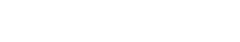#C

The random colors are generated using a JavaScript method - `Math.ceil(Math.random()`* 0xFFFFFF).toString(16)`. Also, since the value of the cell is the same as the color code, you can copy a cell to copy its color code.

``````function colorCodes() {
for (var i = 1; i <= 100; i++) {
sheet.setRowHeight(i, 20);
sheet.setColumnWidth(i, 20);
for (var j = 1; j <= 100; j++) {
var colorcode = Math.ceil(Math.random() * 0xffffff).toString(16);
sheet
.getRange(i, j)
.setBackground('#' + colorcode)
.setValue(color);
}
}
}``````